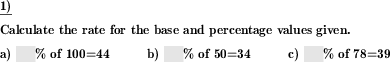Custom math worksheets at your fingertips# Details for problem "Percent rate calculation from base and percentage"

Quickname: 1902

Elementary School, Primary School, Junior High School, Middle School, High School, Senior High School.

## Summary

Base value and percentage are given, calculate the rate and fill in the empty box.

## Example## Description

A series of percentage calculation terms is shown. The rate has been replaced by a blank space.

The base and percentage values are given. The base value is within a choosable number range. It may be a whole number, or a decimal fraction. For the decimal fraction case, the number of decimal places is selectable.

From the values given, the rate has the be calculated and put in the empty box.

The rate is in the 0 to 100 percent range. A raster of 0.1%, 1%, 5% and 10% can be selected for the rate.

The number of problems is selectable.

Download free printable worksheets for this math problem here. The worksheet contains the problems only, the solution sheet includes the answers. Just click on the respective link.

•Worksheet 1Solution sheet with answers
•Worksheet 2Solution sheet with answers
•Worksheet 3Solution sheet with answers

If you can not see the solution sheets for download, they may be filtered out by an ad blocker that you may have installed. If this is the case, please allow ads for this page and reload the page. The solution sheets will then reappear.

• Do these sample worksheets do not really fit?
• Do you need more math worksheets, with a different level of difficulty?
• Would you like to combine different problems on a worksheet and adjust them to your needs?
• As a teacher, you can put together your own worksheets using the automatically generated math problems provided.
With a free initial credit, you can start creating your own math worksheets in a few minutes.

You can try it for free! Register here, to create custom worksheets now!

## Customization options for this problem

Parameter
Possible values
Number of problems
1, 2, 3, 4, 5, 6, 7, 8, 9, 10
Number range
20, 50, 100, 200, 500, 1000, 10000, 100000, 1000000, 10000000
Decimal places
0, 1, 2, 3
Rate raster
0.1%, 1%, 5%, 10%

## Similar problems

Remark
Description
Similar, but calculate the base value
Percent rate and percentage are given, calculate the rate and fill in the empty box.
Mixed questions asking for base, rate or percentage
In a percentage term, one of the base, percentage, or percent rate has to be filled in.
Similar, but calculate the percentage
Calculate percentage from base value and rate.